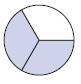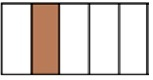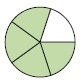# Writing a Decimal and a Fraction for a Shaded Region

Here a figure is given in the form of a circle or a rectangular strip or some other shape. It is then divided into certain number of equal parts. Some of these equal parts are shaded with colors.

Considering the figure as a one unit, it is required to find the fraction that represents the shaded region of the given figure.

It is also required to find the decimal representing the same shaded region.

Following examples will make it easy to write a fraction and a decimal that represent a shaded region in a figure.

Write a decimal or fraction for the shaded region shown below.### Solution

Step 1:

Total parts of the circle = 3

Shaded parts of the circle = 2

Step 2:

Fraction representing shaded region of circle = $\frac{2}{3}$

Step 3:

Writing the fraction as a repeating decimal $\frac{2}{3} = 0.666… = 0.\bar{6}$

Write a decimal or a fraction for the shaded region shown below.### Solution

Step 1:

Total parts of the rectangular strip = 5

Shaded parts of the strip = 1

Step 2:

Fraction representing shaded region of strip = $\frac{1}{5}$

Step 3:

Writing the fraction as a decimal $\frac{1}{5} = \frac{2}{10} = 0.2$

Write a decimal or fraction for the shaded region shown below.### Solution

Step 1:

Total parts of the circle = 5

Shaded parts of the circle = 4

Step 2:

Fraction representing shaded region of circle = $\frac{4}{5}$

Step 3:

Writing the fraction as a decimal $\frac{4}{5} = \frac{8}{10} = 0.8$